# Tension of rope

## Homework Statement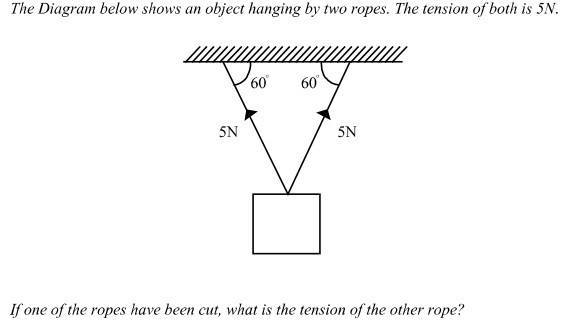## Homework Equations

My assumption:-
if one of the ropes have been cut, it mean the object just hang VERTICALLY with one rope with 5N load on it.Is it my assumption is correct?

## The Attempt at a Solution

Tension of the other rope = 5N (is it correct)?? base on my assumption [/B]

haruspex
Homework Helper
Gold Member
2020 Award
My assumption:-
if one of the ropes have been cut, it mean the object just hang VERTICALLY with one rope with 5N load on it.Is it my assumption is correct?
I would assume they mean the tension at the instant after cutting the other string.

•stuc
phinds
Gold Member
Have you studied force vectors? I think your assumption is half correct and your conclusion is wrong. What you are right about is the hanging vertically. All of your statements about force are wrong.

•stuc
phinds
Gold Member
I would assume they mean the tension at the instant after cutting the other string.
Hm ... could be. I didn't interpret it that way but I guess that could be right. stuc, my comments are not based on that assumption.

•stuc
I would assume they mean the tension at the instant after cutting the other string.
...the tension at the instant after cutting the other string

how to calculate it? it mean my assumption is wrong

i just check an answer..the answer is 5N...but how to get it

haruspex
Homework Helper
Gold Member
2020 Award
Hmm.. that does not fit with either interpretation. But I remain of the view that it's the instantaneous value they're after.
First, can you figure out the weight of the mass?

•stuc
phinds
Gold Member
You have not answered my question about whether or not you have studied force vectors.

•stuc
Hmm.. that does not fit with either interpretation. But I remain of the view that it's the instantaneous value they're after.
First, can you figure out the weight of the mass?
there is no weight given.You have not answered my question about whether or not you have studied force vectors.
learn but always confuse when try to solve it

phinds
Gold Member
there is no weight given.What is the definition of a Newton?

What is the definition of a Newton?
1N = the force needed to accelerate 1kg of mass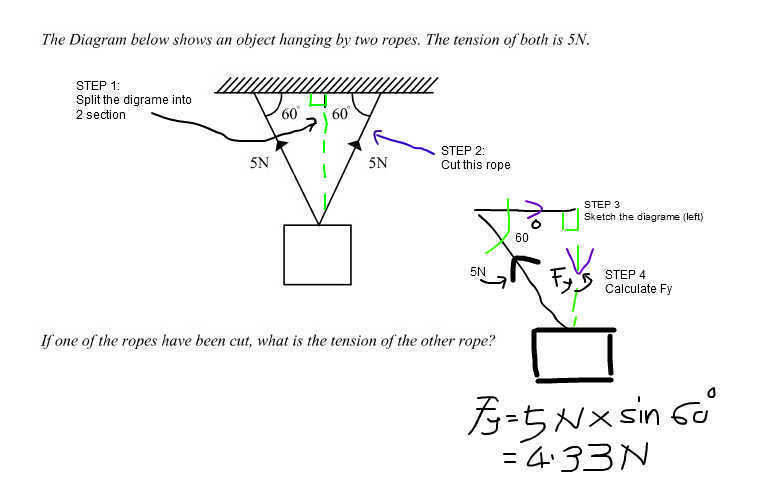Attached is the calculation..any one can verify my ans (4.33N) because the given ans in 5N.

Update:Wrong Arrow for Fy

Last edited: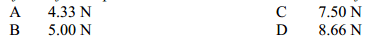Attached other option ans

haruspex
Homework Helper
Gold Member
2020 Award
As I posted, the first step is to determine the weight of the mass. No, it's not given, but there is enough information to find it. To do this, just consider the initial set up with two ropes. Write the equation for balance of forces in the vertical direction.

•stuc
Chestermiller
Mentor
Attached is the calculation..any one can verify my ans (4.33N) because the given ans in 5N.

Update:Wrong Arrow for Fy
You got 4.33 N for the upward component of force contributed by each wire. But there are two wires. So what is the weight of the object? If this weight has to be supported by only one wire, what would the tension in that wire have to be?

Chet

•stuc
As I posted, the first step is to determine the weight of the mass. No, it's not given, but there is enough information to find it. To do this, just consider the initial set up with two ropes. Write the equation for balance of forces in the vertical direction.

My step to determine the weight of the mass..is it ok? (weight of the mass = 7.071N)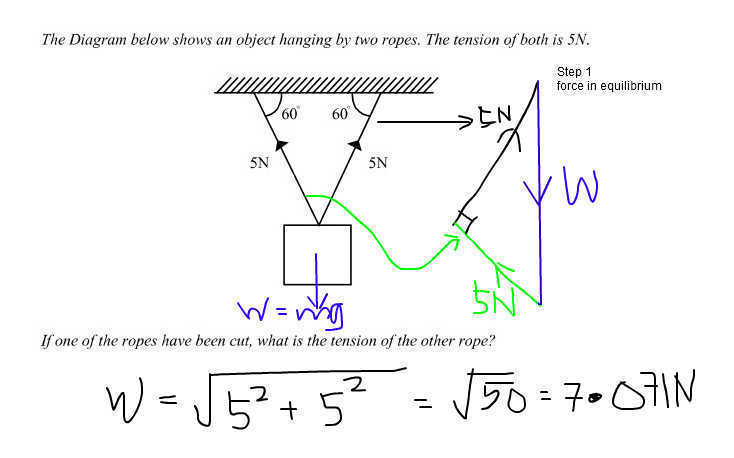You got 4.33 N for the upward component of force contributed by each wire. But there are two wires. So what is the weight of the object? If this weight has to be supported by only one wire, what would the tension in that wire have to be?

Chet
weight of the mass = 7.071Nharuspex
Homework Helper
Gold Member
2020 Award
My step to determine the weight of the mass..is it ok?
View attachment 91455
No, that doesn't work. Pythagoras applies when two vectors at right angles are to be added.
What is the component of each tension in the vertical direction?

•stuc
No, that doesn't work. Pythagoras applies when two vectors at right angles are to be added.
What is the component of each tension in the vertical direction?..tq for the info..will be calculate

haruspex
Homework Helper
Gold Member
2020 Award..tq for the info..will be calculate
Hint: you already calculated it in post #12.

Chestermiller
Mentor
My step to determine the weight of the mass..is it ok? (weight of the mass = 7.071N)
View attachment 91455
In this figure, the 5 N should be the hypotenuse, not one of the other sides. You're using the wrong triangle.

•stuc
Hint: you already calculated it in post #12.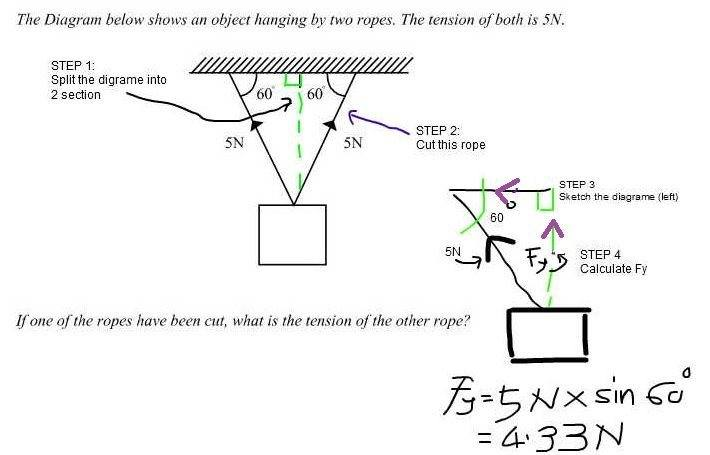Base on the above diagram (arrow edited),

5N x sin 60 + 5N x sin 60 - W = 0
therefore W= 2(5N x sin 60)
= 8.66 N

You got 4.33 N for the upward component of force contributed by each wire. But there are two wires. So what is the weight of the object? If this weight has to be supported by only one wire, what would the tension in that wire have to be?

Chet
i get W=8.66N ..but how the answer is 5Nharuspex
Homework Helper
Gold Member
2020 Award
i get W=8.66N ..but how the answer is 5NSteady on, so far all we have found is the hanging weight. The tension in one string immediately after the other is cut might be different. So leave the weight in the form ##10\sin(60)N## for now.
Now draw a free body diagram for the weight just after one string is cut. What equation can you obtain for the tension in the remaining string.

Steady on, so far all we have found is the hanging weight. The tension in one string immediately after the other is cut might be different. So leave the weight in the form ##10\sin(60)N## for now.
Now draw a free body diagram for the weight just after one string is cut. What equation can you obtain for the tension in the remaining string.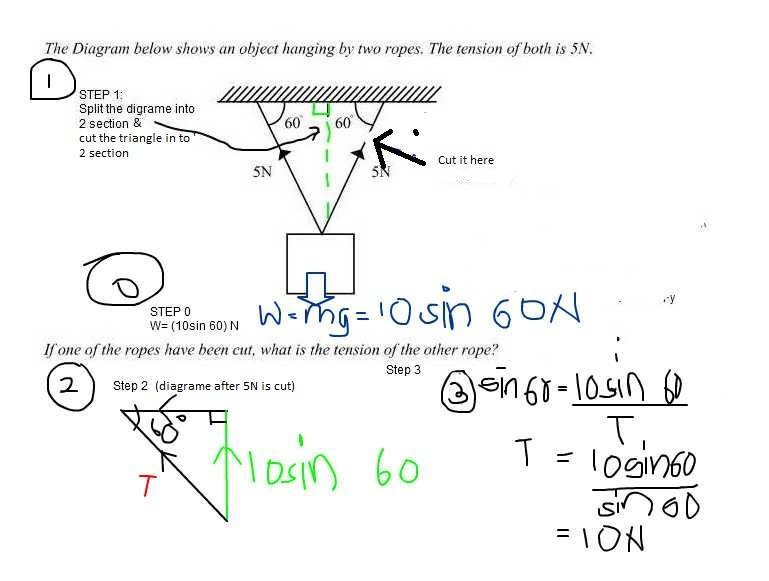the ans is 10N after cut the 5N right rope...huhu near but still cannot get the ans